Custom Search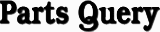VOLTAGE MULTIPLIERS You may already know how a transformer functions to increase or decrease voltages. You may also have learned that a transformer secondary may provide one or several ac voltage outputs which may be greater or less than the input voltage. When voltages are stepped up, current is decreased; when voltages are stepped down, current is increased. Another method for increasing voltages is known as voltage multiplication. VOLTAGE MULTIPLIERS are used primarily to develop high voltages where low current is required. The most common application of the high voltage outputs of voltage multipliers is the anode of cathode-ray tubes (CRT), which are used for radar scope presentations, oscilloscope presentations, or TV picture tubes. The dc output of the voltage multiplier ranges from 1000 volts to 30,000 volts. The actual voltage depends upon the size of the CRT and its equipment application. Voltage multipliers may also be used as primary power supplies where a 177 volt-ac input is rectified to pulsating dc. This dc output voltage may be increased (through use of a voltage multiplier) to as much as 1000 volts dc. This voltage is generally used as the plate or screen grid voltage for electron tubes. If you have studied transformers, you may have learned that when voltage is stepped up, the output current decreases. This is also true of voltage multipliers. Although the measured output voltage of a voltage multiplier may be several times greater than the input voltage, once a load is connected the value of the output voltage decreases. Also any small fluctuation of load impedance causes a large fluctuation in the output voltage of the multiplier. For this reason, voltage multipliers are used only in special applications where the load is constant and has a high impedance or where input voltage stability is not critical. Voltage multipliers may be classified as voltage doublers, triplers, or quadruplers. The classification depends on the ratio of the output voltage to the input voltage. For example, a voltage multiplier that increases the peak input voltage twice is called a voltage doubler. Voltage multipliers increase voltages through the use of series-aiding voltage sources. This can be compared to the connection of dry cells (batteries) in series. The figures used in the explanation of voltage multipliers show a transformer input, even though for some applications a transformer is not necessary. The input could be directly from the power source or line voltage. This, of course, does not isolate the equipment from the line and creates a potentially hazardous condition. Most military equipments use transformers to minimize this hazard. Figure 4-44 shows the schematic for a half-wave voltage doubler. Notice the similarities between this schematic and those of half-wave voltage rectifiers. In fact, the doubler shown is made up of two half-wave voltage rectifiers. C1 and CR1 make up one half-wave rectifier, and C2 and CR2 make up the other. The schematic of the first half-wave rectifier is indicated by the dark lines in view A of figure 4-45. The dotted lines and associated components represent the other half-wave rectifier and load resistor. Figure 4-44. - Half-wave voltage doubler.Figure 4-45A. - Rectifier action of CR1 and CR2. POSITIVE ALTERNATION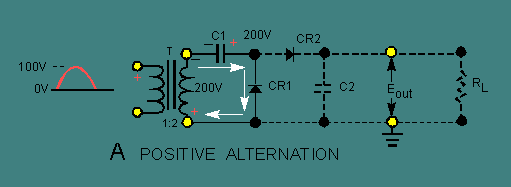Notice that C1 and CR1 work exactly like a half-wave rectifier. During the positive alternation of the input cycle (view A), the polarity across the secondary winding of the transformer is as shown. Note that the top of the secondary is negative. At this time CR1 is forward biased (cathode negative in respect to the anode). This forward bias causes CR1 to function like a closed switch and allows current to follow the path indicated by the arrows. At this time, C1 charges to the peak value of the input voltage, or 200 volts, with the polarity shown. During the period when the input cycle is negative, as shown in view B, the polarity across the secondary of the transformer is reversed. Note specifically that the top of the secondary winding is now positive. This condition now forward biases CR2 and reverse biases CR1. A series circuit now exists consisting of C1, CR2, C2, and the secondary of the transformer. The current flow is indicated by the arrows. The secondary voltage of the transformer now aids the voltage on C1. This results in a pulsating dc voltage of 400 volts, as shown by the waveform. The effect of series aiding is comparable to the connection of two 200-volt batteries in series. As shown in figure 4-46, C2 charges to the sum of these voltages, or 400 volts. Figure 4-45B. - Rectifier action of CR1 and CR2. NEGATIVE ALTERNATION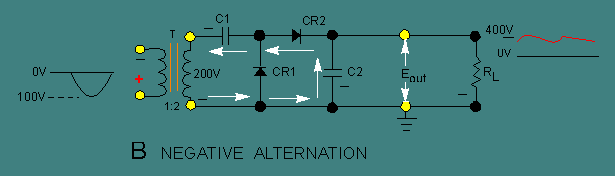Figure 4-46. - Series-aiding sources.The schematic shown in figure 4-47 is an illustration of a half-wave voltage tripler. When you compare figures 4-46 and 4-47, you should see that the circuitry is identical except for the additional parts, components, and circuitry shown by the dotted lines. (CR3, C3, and R2 make up the additional circuitry.) By themselves, CR3, C3, and R2 make up a half-wave rectifier. Of course, if you remove the added circuitry, you will once again have a half-wave voltage doubler. Figure 4-47. - Half-wave voltage tripler.View A of figure 4-48 shows the schematic for the voltage tripler. Notice that CR3 is forward biased and functions like a closed switch. This allows C3 to charge to a peak voltage of 200 volts at the same time C1 is also charging to 200 volts. Figure 4-48A. - Voltage tripler. POSITIVE ALTERNATION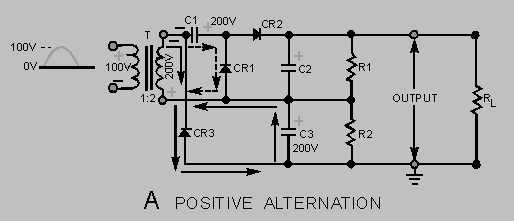The other half of the input cycle is shown in view B. C2 is charged to twice the input voltage, or 400 volts, as a result of the voltage-doubling action of the transformer and C1. At this time, C2 and C3 are used as series-aiding devices, and the output voltage increases to the sum of their respective voltages, or 600 volts. R1 and R2 are proportional according to the voltages across C2 and C3. In this case, there is a 2 to 1 ratio. Figure 4-48B. - Voltage tripler. NEGATIVE ALTERNATIONThe circuit shown in figure 4-49 is that of a full-wave voltage doubler. The main advantage of a full-wave doubler over a half-wave doubler is better voltage regulation, as a result of reduction in the output ripple amplitude and an increase in the ripple frequency. The circuit is, in fact, two half-wave rectifiers. These rectifiers function as series-aiding devices except in a slightly different way. During the alternation when the secondary of the transformer is positive at the top, C1 charges to 200 volts through CR1. Then, when the transformer secondary is negative at the top, C2 charges to 200 volts through CR2. R1 and R2 are equal value, balancing resistors that stabilize the charges of the two capacitors. Resistive load RL is connected across C1 and C2 so that RL receives the total charge of both capacitors. The output voltage is +400 volts when measured at the top of R L, or point "A" with respect to point "B." If the output is measured at the bottom of RL, it is -400 volts. Either way, the output is twice the peak value of the ac secondary voltage. As you can imagine, the possibilities for voltage multiplication are extensive. Figure 4-49. - Full-wave voltage doubler.Q.38 A half-wave voltage doubler is made up of how many half-wave rectifiers?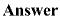Q.39 If a half-wave rectifier is added to a half-wave voltage doubler, the resulting circuit is a voltage ____.Q.40 In a full-wave voltage doubler, are the capacitors connected in series or in parallel with the output load?Integrated Publishing, Inc. - A (SDVOSB) Service Disabled Veteran Owned Small Business